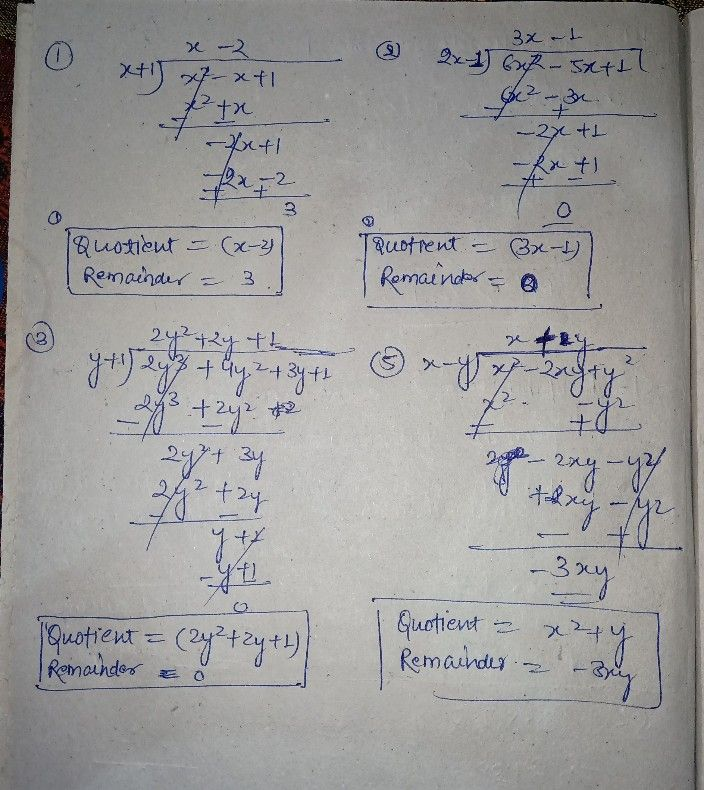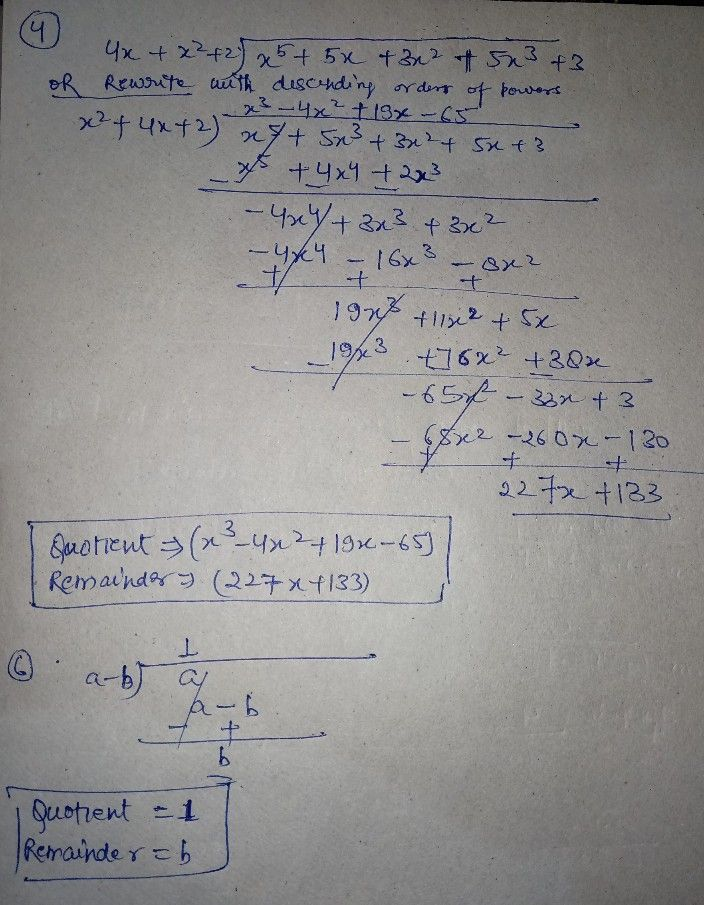Symbol
ProblemFind the quotient and remainder on dividing polynomial $x^{2}-x+1byx+1$ Find the quotient and remainder on dividing $6x^{2}-5x+1$ $by2x-1$ Find the quotient and remainder on dividing $2y^{3}+4y^{2}+3y+1byy+1$ Find the quotient and remainder on dividing $x^{3}+5x+3x^{2}+5x^{3}+3$ b $4x+x^{2}+2$ Find the quotient and remainder on dividing $x^{2}-2xy+y^{2}byx-y$ Divide polynomial a by polynomial $a-b$
10th-13th grade
Other
Search count: 115
SolutionQanda teacher - Rahulrajpt